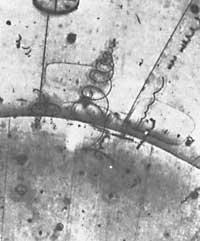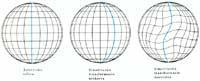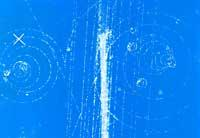# In the bowels of nature: symmetries

1986/10/01 Martinez Lizarduikoa, Alfontso Iturria: Elhuyar aldizkaria

Any phenomenon that participates in the framework of nature can be explained according to four basic forces: They are weak nuclear force, strong nuclear force, electromagnetic force and gravitational force. Each force has its scale of distances in which its influence predominates. Gravitation predominates on the cosmic scale, but today physicists, even on the ultramicroscopic scale (Planck scale), consider that gravitation has a very important behavior. The study of gravitation on this scale has given rise to a new branch of physics: Quantum gravitation.

## Unified zone and fractions acceleratorsThis Standfor accelerator (California) produces electrons and positrons and in its elimination are born lots of new fractions.

In the four basic forces that physicists recognize to explain phenomena that occur in nature, gravity is the first that was historically created and Newton was their inventor. By this force the relationship between material fractions is structured. In Newtonian physics, matter (material fraction) is the only element of actual existence in the preposed space-time framework.

However, in the 1850s Maxwell, mathematically Faraday's work, built a new independent physical theory of gravitation: Electromagnetic. In this new theory, Electricity and Magnetism were defined as different aspects of a common phenomenon, such as vibrations of the electromagnetic field.

If in Newton's metaphysics the fraction appears as an irreducible element, Maxwell's metaphysics is based on the field. From this moment on all science will appear submerged in the fraction/wave duality, and that duality is still alive today.

To overcome this fraction of nature/power identity, Einstein tried to build the theory of common space. But in vain. Mechanics and Electromagnetism, obstinate, will maintain independence from each other, appearing Nature in its bowels as dualist (and not monist).The length of this accelerator at the stand (California) is 3 km.

However, to this day the desire has come to achieve the unity of all spheres, that is, to show the universe by one force. Today, physicists, with the help of mathematicians, are pursuing this goal with more enthusiasm than ever, and proof of this are the giant accelerators of fractions built and under construction. This task has not been similar in the history of science, and to build these machines enormous economic and social cooperation between states is needed. (1).

## Quantum electrodynamics

On the road to a unified field, the last period of 1960-70 years was very important, since in those years quantum mechanics adapted very successfully to electromagnetic fields. From this union came quantum electrodynamics. During the fifteen years in which this theory has existed, it has demonstrated enormous precision and ability to predict phenomena.

Thanks to this incomparable success, physicists began to quantify three other forces in nature. But immediately they began to resign. While violent force and weak force were not well understood, the theory of quarks (quantum chromodynamics) was not consolidated, and gravitation escaped again and again to quantization. About fifteen years ago the situation was as follows: To express the four forces he had in nature, there were four different theories, of which only one (quantum electrodynamics) worked from the point of view of the scientific method.

A constant question was asked about the researchers. What was the specific structure of the electromagnetic field in order to give such adequate and precise quantum representation to the other three fields? If we explain the internal structure of the electromagnetic field, would it not be possible to adapt this structure to the other three zones thus constructing the unified field theory?

## Maxwell electromagnetic and symmetries

Since the birth of human civilization, the influence of symmetrical models on thought and religion is undeniable, especially symmetrical forms. But the concept of symmetry is very deep and not just formal symmetry. There is a very broad field, still unexplored: the most abstract symmetry. This field of abstract symmetries has been the quarry of modern physics. And its importance lies in the fact that today's physicists believe that the existence of the four basic forces that appear in nature is given to perpetuate certain symmetries that reside in the simplest structure of nature.The relationship between physics and geometric symmetry has long been known by subsistence laws. The laws of permanence tell us that something remains constant over time. The easiest way to see the symmetries are the rotacias and the reflections, but not all the types of symmetries that explains Nature.

Sometimes, when analyzing the mathematical description of a physical system it is possible to find new types of symmetry. We have a historically very representative example: Maxwell electromagnetic field equations. Maxwell, analyzing his equations, discovered that electrical and magnetic components did not appear fully symmetrical. Under a deep sense of aesthetic intuition, he added a new theme in his equations to be symmetrical. This new component managed to represent the magnetic field that produces a variable electric field, the new effect, which was experimentally detected.

However, it took more than 50 years to understand the depth of symmetries in Maxwell's equations. Lorentz and Poincaré discovered with surprise that Maxwell's equations were symmetrical with respect to a summing operation of spaces and times. If we associate time with the three spatial dimensions, the structure in four dimensions is obtained space-time, then the symmetry of Lorentz/Poincaré is space-time rotation. That is, there is a deep relationship between electromagnetism and space/time; space/time deforms symmetrically when the observer approaches the speed of light. Taking as real this phenomenon so far from traditional experience, Einstein clarified a new era: The era of relativity.Rotation is a symmetry that maintains the invariable shape of the sphere. In addition, symmetry is general because all the points on its surface form the same angular displacement. Reduced symmetry requires maintaining the unalterable shape of the sphere, although the points of the surface of the sphere move independently. Reduced symmetry strains the sphere and generates forces at points.

This trajectory throughout the history of science warns us of the importance of mathematical symmetries to explain the hidden foundations that exist in nature.

## Renormalization and gauge transformation

One of the most important obstacles presented to them when conducting theoretical research on the part of current physicists is the infinite terms; in the equations that use them, often, the infinite values that appear in physical magnitudes. Faced with this serious obstacle, the researcher has two ways: abandoning the model used (which means returning from nowhere) or finding a solution to overcome it. Logically, physicists have opted for this second way, and when these infinite ones appear, they renormalize the origin of the scale (corresponding to the zero point) used by them so that the calculation can continue, disappearing infinite quantities. Through it theorists discard infinite values.

Quantum electrodynamics is a theory that can be renormalized. But when we want to reinormalize the other three forces of Nature we see that it is not possible. Therefore, theoretical physicists began to think that the secret of the success of quantum electrodynamics remained in its internal structure, and that its discovery would be applicable to other fields (forces).

It immediately begins to be suspected that this internal structure was symmetry, and the conviction that for a theory to be stable needed a special internal symmetry spread rapidly in scientific environments. Therefore, some possible special symmetries at the origin of the four forces were immediately analyzed: The so-called “gauge”. (2)

Gauge symmetries are closely related to the renormalization problem. These symmetries allow to reestimate the scale of some physical magnitude. If a system or theory keeps invariant the physical character of this system or theory regarding a exchange rate, it will have Gauge symmetry.Creation of antimatter. Particles, with electric charge, become visible in liquid helios because they leave a trail of bubbles. The antimatery produced in this case is positron. The spiral that creates it is clockwise and occupies two-thirds of the right of the image. The small spiral is a direction electron contrary to the clock hands. Positron is the antiparticle of the electron.

It is possible to reestimate the scale of a magnitude throughout the field of analysis; then we are facing a global transformation of Gauge. If the scale changes are different at different points in the area when it comes to normalization, the Gauge transformation is reduced.

## Gravitation and symmetries of nature

We will return to nature and analyze the controversial and mysterious phenomenon of gravitation. Suppose a laboratory is located in a spatial concentration and that this laboratory is moving through space in a straight line, where there are no external forces. Now suppose the second case. In this case the laboratory is moving through the space, but its route is curved

accelerated therefore

Moving from the first to the second case is possible, and for this we only have to introduce the influence of an external field. More specifically, introduce a gravitational field.

The trajectory of a laboratory in the orbit of a planet is the same as that of a laboratory when moving through empty space. And the reason is simple. The curved path in an orbit compensates the forces it exerts on the laboratory by gravity. From this point of view, gravity is a compensatory field by which the deviation of the system from the straight line is recovered.

In this sense, if the introduced gravitational field were sufficiently complex, we could obtain symmetrical physical laws, even if we had reduced gauge transformations. The gravitational field would be the behavior of Nature to keep gauge symmetry reduced. In this case, the content of symmetry would be the unalterability of physical laws when the course of a movement changes arbitrarily.

If we analyze physical phenomena from this point of view, gravity could be redefined as the appearance of symmetry in Nature.Fermilab accelerator viscera.

Thus things, the four basic forces that govern the behavior of the universe would be the compensatory areas that nature creates to balance the reduced night symmetries in the internal structure. In turn, the force fields will be the way to impose on the activity of nature the reduced gauge symmetries in the universe.

If we review the most general physical constructions from this perspective, we will realize that the electromagnetic field is the easiest night symmetry to match with reduced relativity. In this case it would correspond to changes in voltaic gauge transformations.

It has been very important to note that the problem of renormalization and night symmetry are deeply intertwined. In short, the superperfect operation of quantum electrodynamics and its capacity for renormalization lies in the simple internal structure of the electromagnetic field.

OBSERVATIONS:

Among the current fraction accelerators, the FERMILAB (Fermi National Accelerator Laboratory) is one of the most powerful. This machine accelerates the Protons, whose beam energy can reach 1000 GeV. By 1990 the accelerator called UNK will be launched in the USSR, obtaining an energy of 3000 GeV. Around these dates the new FERMILAB of 5000 GeV will be launched. Finally, it is worth mentioning that it is the largest technological project that has been proposed in human existence. This project is currently at the design level. This accelerator is called VBA (Very Big Accelerator) and accelerates protons. The energy this machine could get would be 20,000 GeV. To build such a device it will be necessary to collaborate with different States. We still do not know the year of its implementation, but being so giant, the only appropriate place for its construction will be the desert. And there they will build it. In English gauge symmetries . In Spanish, gauge symmetry or capacity symmetry.eu es fr en ca gl

## Gai honi buruzko eduki gehiago

Elhuyarrek garatutako teknologia

1987-2-1Zientzia
##### Indar kosmikoen batasuna
1986-12-1Zientzia